﻿ Dirichlet Problem: Articles, Studies and Background Information
Dirichlet Problem
Articles, Studies and Background Information

Resources

### Background Information

• Dirichlet problem - Wikipedia [View Resource]
• Dirichlet Problem - MathWorld [View Resource]
• Dirichlet problem [View Resource]

### K-12 Science Fair Projects

• The Solution of the Dirichlet Problem with Rational Boundary Data [View Resource]

### Undergraduate Lesson Plans, Studies and Articles

• Solving Dirichlet problem for a circle and a half plane-The Poisson integral formula [View Resource]
• Fourier analysis and the Dirichlet problem [View Resource]
• The Dirichlet Problem on Quadratic Surfaces [View Resource]
• Solvability Of The Dirichlet Problem In Sobolev Space Coercivity And Garding's Inequality [View Resource]
• Geometric aspects of the Dirichlet problem [View Resource]
• Dirichlet's Problem on The Disk [View Resource]
• Dirichlet Duality and the Nonlinear Dirichlet Problem [View Resource]
• The Dirichlet Problem: solution by simple formula by complex variable methods [View Resource]
• Lesson: The Dirichlet Problem in an Annulus [View Resource]
• Brownian Motion And Dirichlet Problems At Infinity [View Resource]
• Optimized Schwarz Methods for the Advection-Diffusion Equation [View Resource]

### Theses and Dissertations

• The Schrodinger Equation as a Volterra Problem [View Resource]
• Analysis of conduction heat transfer in semi-infinite slabs and infinite quadrants with discrete heat generation sources using Green’s function integral methods [View Resource]
• On the Isoperimetric Problem for the Laplacian with Robin and Wentzell Boundary Conditions [View Resource]
• Topics in the asymptotic analysis of linear and nonlinear eigenvalue problems [View Resource]
Background Information

## Definition

The Dirichlet problem is the problem of finding a function which solves a specified partial differential equation (PDE) in the interior of a given region that takes prescribed values on the boundary of the region.

## General solution

For a domain D having a sufficiently smooth boundary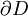, the general solution to the Dirichlet problem is given by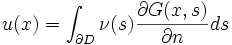where G(x,y) is the Green's function (used to solve inhomogeneous differential equations subject to specific initial conditions or boundary conditions) for the partial differential equation, and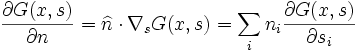is the derivative of the Green's function along the inward-pointing unit normal vector. The integration is performed on the boundary, with measure ds. The function ν(s) is given by the unique solution to the Fredholm integral equation of the second kind,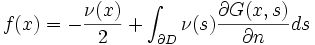.

The Green's function to be used in the above integral is one which vanishes on the boundary:

G(x,s) = 0

forand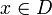.

Such a Green's function is usually a sum of the free-field Green's function and a harmonic solution to the differential equation.

### Existence

The Dirichlet problem for harmonic functions always has a solution, and that solution is unique, when the boundary is sufficiently smooth and f(s) is continuous. More precisely, it has a solution when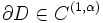for 0 < α, where C(1,α) denotes the Hölder condition (a real or complex-valued function ƒ on d-dimensional Euclidean space satisfies a Hölder condition when there are nonnegative real constants).

Source: Wikipedia (All text is available under the terms of the GNU Free Documentation License and Creative Commons Attribution-ShareAlike License.)

Follow Us On: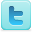Privacy Policy - Site Map - About Us - Letters to the Editor

Comments and inquiries could be addressed to:
webmaster@julianTrubin.com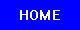Last updated: June 2013
Copyright © 2003-2013 Julian Rubin